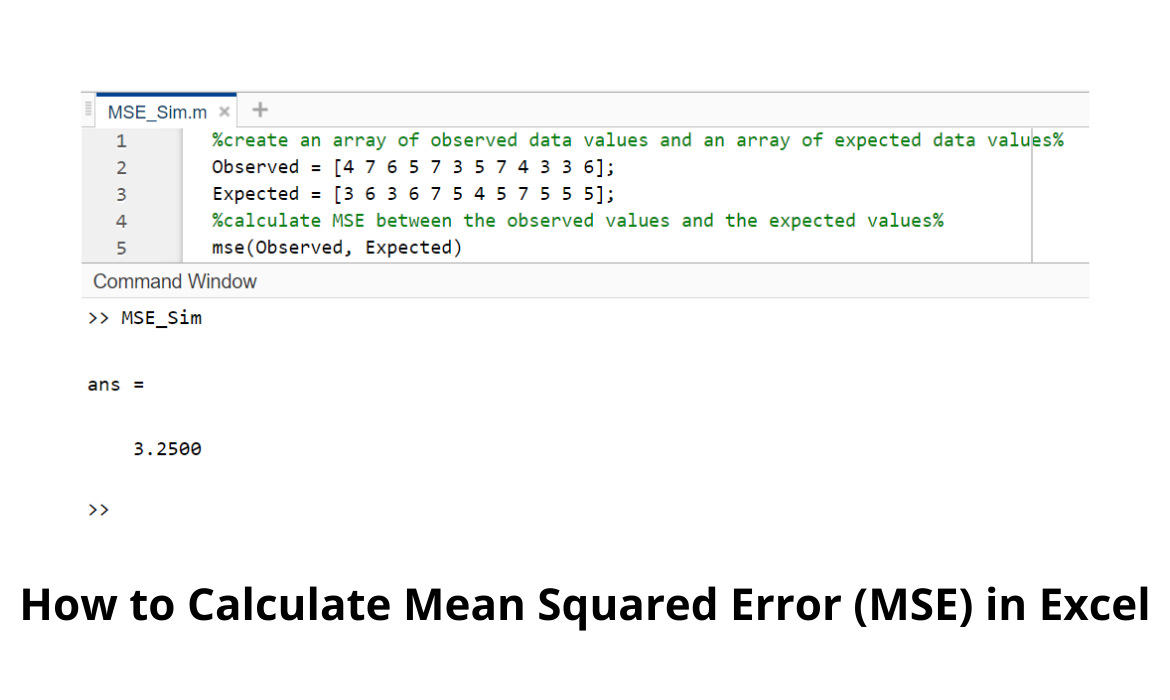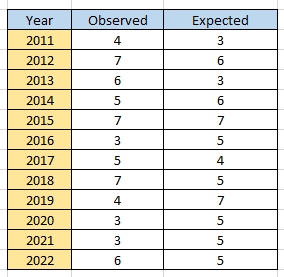# How to Calculate Mean Squared Error (MSE) in ExcelMean Squared Error (MSE) is one of the most routine parameters that is used for measuring the forecast accuracy of a model.

In layman’s terms, it is the mean of the squares of the error margin between observed and expected values.

The formula for MSE is:

MSE = (1/N) * Σ(O – E)2

Where:

Σ stands for summation or sum

N = sample size

O = observed data value (or actual value)

E = expected data value (or forecasted value)

Mean squared error gives a measure of the closeness of a regression line to a set of points.

It achieves this using the squares of the distances between the points and the regression line.

The squaring helps to eliminate negative signs.

As a rule of thumb, the MSE value is inversely proportional to the accuracy level of the forecast model.

This implies that a lower MSE value will result in a more accurate forecast model and vice-versa.

## How to Calculate Mean Squared Error (MSE) in Excel

It’s pretty easy and straightforward.

In literally three steps, you’re done!

In this short tutorial, we’ll calculate the mean square error between the actual and forecasted number of cars (in thousands) manufactured by company X between 2011 and 2022.

### Step 1: Set up the table of values

First, we arrange the observed and expected values in adjacent columns as shown below:### Step 2: Calculate the “squared error” for each entry.

From the initial formula provided for MSE in the introductory part of this article, the term (O – E)2 is known as the squared error.

We will use this formula to add an extra column to our table of values:When we cascade this to all the rows of the table, the resulting table is as shown below:### Step 3: Calculate the mean squared error

Next, we calculate the mean squared error by simply finding the arithmetic mean (or average) of the squared errors, using the “AVERAGE” function in excel.The resulting MSE of cars manufactured by company X between 2011 and 2022 is 3.25 as shown in the final table below:This result can be verified using MATLAB, by using the mse(O, E) function

Where:

• O = observed data value (or actual value)
• E = expected data value (or forecasted value)

The same example can be modelled by creating an array of both observed and expected values, and then applying the mse(O, E) function to calculate the MSE between the observed values and the expected values.

The resulting MSE of cars manufactured by company X between 2011 and 2022 is 3.25 as shown in the MATLAB model below.## Summary & Conclusion

In this brief article, we have outlined what mean square error (MSE) is, its formula and its uses.

We stated that it is used to assess the accuracy of a model.

We also saw an example of how to compute MSE by calculating the mean square error of cars manufactured by a fictitious car company – company X.

In addition to MSE, other metrics that can be used to assess the accuracy of a model are mean absolute deviation (MAD) and mean absolute percentage error (MAPE).

Ovo is a highly specialized Computer and Networking Expert with experience in Windows, Cisco, Microsoft Office (Excel, Word, etc) and Networking engineering. Ovo is a creative, team player that loves sharing his experience with technology with readers to follow along. He has great attention to detail when discussing various technologies, tutorials and guides.